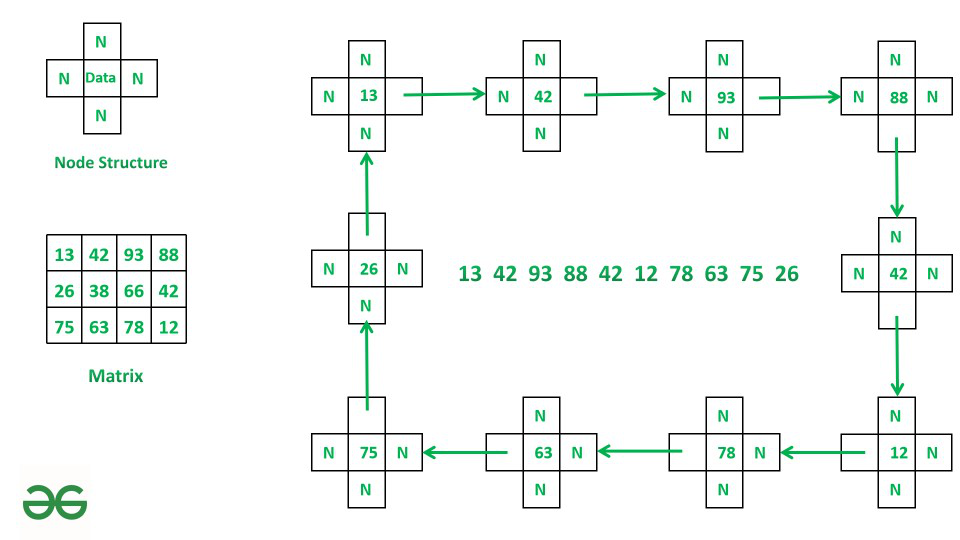# Form a Rectangle from boundary elements of Matrix using Linked List

• Difficulty Level : Hard
• Last Updated : 06 Oct, 2021

Given a Matrix grid[][] of size NxM where N is number of rows and M is number of columns. The task is to form a rectangle from boundary elements of grid[][] using linked list having four pointers namely prev, next, top and bottom. Print the final linked list.

Examples:

Attention reader! Don’t stop learning now. Get hold of all the important DSA concepts with the DSA Self Paced Course at a student-friendly price and become industry ready.  To complete your preparation from learning a language to DS Algo and many more,  please refer Complete Interview Preparation Course.

In case you wish to attend live classes with experts, please refer DSA Live Classes for Working Professionals and Competitive Programming Live for Students.

Input: A = [[13, 42, 93, 88],
[26, 38, 66, 42],
[75, 63, 78, 12]]
Output: 13 42 93 88 42 12 78 63 75 26

Explanation:1. Make A and head node
2. Traverse through 0th row and create node for each element and connect them through next pointer.
3. Traverse through (m-1)th column and create node for each element and connect them through bottom pointer.
4. Traverse through (n-1)th row and create node for each element and connect them through prev pointer.
5. Traverse through 0th column and create node for each element and connect them through top pointer.
6. Step 2, 3, 4, 5 is repeated till temp. The top become equal to head.

Input: A = [[1, 2, 3]
[8, 9, 4]
[7, 6, 5]]
Output: 1 2 3 4 5 6 7 8

Approach: This problem can be solved by Performing boundary traversal of matrix and creating nodes for each element and link them using next, prev, bottom or top and create a linked list.

Step 1: Make grid as the head of the Linked list and initialize temp as the head.
Step 2: Traverse through the first row from j=1 to j=m-1 where i=0 and create a node for each element and link them through the next pointer.
Step 3: Traverse through the last column from i=0 to i=n-1 where j=m-1 and create a node for each element and link them through a bottom pointer.
Step 4: Traverse through the last row from j=m-1 to j=0 where i=n-1 and create a node for each element and link them through the prev pointer.
Step 5: Traverse through the first column from i=n-1 to i=0 where j=0 and create a node for each element and link them through the top pointer.
Step 6: Step 2, 3, 4, 5 is repeated till temp.top becomes equal to head.
Step 7: Print the required Linked List.

Below is the implementation of the above algorithm.

## Python3

 `# Python program for above approach``# Node Class``class` `Node:` `    ``# Constructor to initialize the node object``    ``def` `__init__(``self``, val):``        ``self``.data ``=` `val``        ``self``.``next` `=` `None``        ``self``.prev ``=` `None``        ``self``.top ``=` `None``        ``self``.bottom ``=` `None`  `# Linked List class``class` `LinkedList:` `    ``# Constructor to initialize head``    ``def` `__init__(``self``):``        ``self``.head ``=` `None` `    ``# function to form square``    ``# linked list of matrix.``    ``def` `Quad(``self``, grid, n, m):` `        ``# initialising A as head.``        ``self``.head ``=` `Node(grid[``0``][``0``])` `        ``# head is assigned to head.``        ``temp ``=` `self``.head` `        ``# i is row index, j is column index``        ``i ``=` `0``        ``j ``=` `1` `        ``# loop till temp.top become equal to head.``        ``while` `temp.top !``=` `self``.head:``            ``# as we iterating over boundary``            ``# of matrix so we will iterate``            ``# over first(0) and last(n-1) row``            ``# and first(0) and last(m-1) column.` `            ``# iterating over first i.e 0th row``            ``# and connecting node.``            ``if` `j < m ``and` `i ``=``=` `0``:``                ``temp.``next` `=` `Node(grid[i][j])``                ``temp ``=` `temp.``next``                ``j ``+``=` `1` `            ``# iterating over last i.e (m-1)th``            ``# column and connecting Node.``            ``elif` `j ``=``=` `m ``and` `i < n ``-` `1``:``                ``i ``=` `i ``+` `1``                ``temp.bottom ``=` `Node(grid[i][j ``-` `1``])``                ``temp ``=` `temp.bottom` `            ``# iterating over last i.e (n-1)th row``            ``# and connecting Node.``            ``elif` `i ``=``=` `n ``-` `1` `and` `j <``=` `m ``and` `j >``=` `1``:``                ``if` `j ``=``=` `m: j ``=` `j ``-` `1``                ``j ``=` `j ``-` `1``                ``temp.prev ``=` `Node(grid[i][j])``                ``temp ``=` `temp.prev` `            ``# iterating over first i.e 0th column``            ``# and connecting Node.``            ``elif` `i <``=` `n ``-` `1` `and` `j ``=``=` `0``:``                ``i ``=` `i ``-` `1``                ``temp.top ``=` `Node(grid[i][j])``                ``temp ``=` `temp.top``                ``if` `i ``=``=` `1``:``                    ``temp.top ``=` `self``.head` `    ``# function to print Linked list.``    ``def` `printList(``self``, root):``        ` `        ``temp ``=` `root``        ` `        ``# printing head of linked list``        ``print``(temp.data, end ``=``" "``)``        ` `        ``# loop till temp.top``        ``# become equal to head``        ``while` `temp.top !``=` `root:``          ` `          ``# printing the node``            ``if` `temp.``next``:``                ``print``(temp.``next``.data, end ``=``" "``)``                ``temp ``=` `temp.``next``            ``if` `temp.prev:``                ``print``(temp.prev.data, end ``=``" "``)``                ``temp ``=` `temp.prev``            ``if` `temp.bottom:``                ``print``(temp.bottom.data, end ``=``" "``)``                ``temp ``=` `temp.bottom``            ``if` `temp.top:``                ``print``(temp.top.data, end ``=``" "``)``                ``temp ``=` `temp.top` `# Driver Code``grid ``=` `[[``13``, ``42``, ``93``, ``88``],   ``        ``[``26``, ``38``, ``66``, ``42``],``        ``[``75``, ``63``, ``78``, ``12``]]` `# n is number of rows``n ``=` `len``(grid)` `# m is number of column``m ``=` `len``(grid[``0``])` `# creation of object``l ``=` `LinkedList()` `# Call Quad method to create Linked List.``l.Quad(grid, n, m)` `# Call printList method to print list.``l.printList(l.head)`

## Javascript

 ``
Output
`13 42 93 88 42 12 78 63 75 26 `

Time Complexity: O(N*M)
Auxiliary Space: O(N*M)

My Personal Notes arrow_drop_up